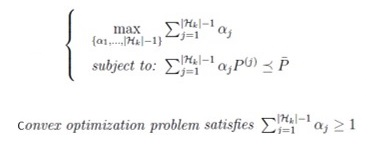# Cvx code for convex optimization problem

Hi guys, I am new in matlab and cvx and need your help. I went through the cvx guide and tried to come up with the matlab code for this optimization problem.
I am trying to code the convex optimization problem below using cvx and I’m getting an error when using “alpha” as a variable.
Tried to use “X” as a variable and had a different error. Should I have to use a different syntax? Please advise.#Code using “alpha” as variable:

H=4
P_bar=randn(n,n)
j=4
P=randn(n,n)
cvx_begin
variable alpha(n,n) symetric
minimize(-sum(alpha))
subject to
for j=1;abs(H)-1,
sum(alpha*P(:,j)) <= P_bar
X(:,j)>=0
end
cvx_end

H =

 4


P_bar =

0.7283   -1.3573
2.1122   -1.0226


j =

 4


P =

1.0378   -1.3813


-0.3898 0.3155

Error using variable (line 100)
Variable name “alpha” is the name of an existing MATLAB function or directory:
C:\Program Files\MATLAB\R2017b\toolbox\matlab\graph3d\alpha.m

#Code using “X” as variable:

H=4
P_bar=randn(n,n)
j=4
P=randn(n,n)
cvx_begin
variables X(n,n) symetric
minimize(-sum(X))
subject to
for j=1;abs(H)-1,
sum(X*P(:,j)) <= P_bar
X(:,j)>=0
end
cvx_end

H =

 4


P_bar =

1.1902    0.6353


-1.1162 -0.6014

j =

 4


P =

0.5512    0.0860


-1.0998 -2.0046

ans =

 3


Error using cvx_end (line 267)
Your objective function is not a scalar.

Your second code makes no sense at all.

In your first code, alpha should be a vector. i.e.,variable alpha(n,1) . You should use cvx_begin sdp (either that or use == semdefinite(n) form). The LMI makes no sense unless P_bar is symmetric Your LMI should be summing.product of alpha component times a symmetric matrix

But actually, CVX s telling you not to use the variable name alpha, so change it to something else, such as ALPHA .

Thanks for your help Mr. Mark. I will try that way. Regards